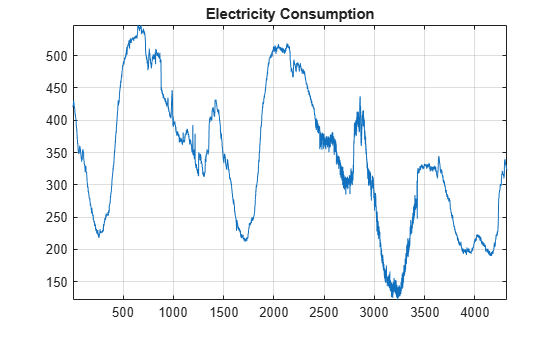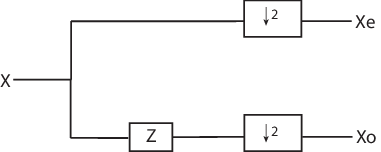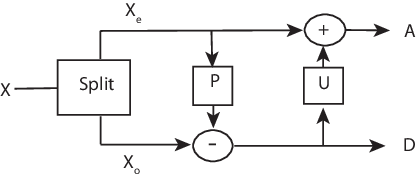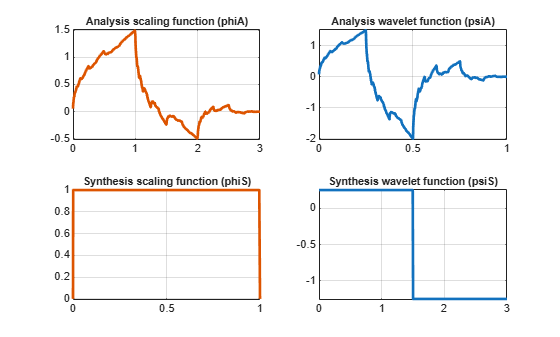## Lifting Method for Constructing Wavelets

The so-called first generation wavelets and scaling functions are dyadic dilations and translates of a single function. Fourier methods play a key role in the design of these wavelets. However, the requirement that the wavelet basis consist of translates and dilates of a single function imposes some constraints that limit the utility of the multiresolution idea at the core of wavelet analysis.

The utility of wavelet methods is extended by the design of second generation wavelets via lifting.

Typical settings where translation and dilation of a single function cannot be used include:

• Designing wavelets on bounded domains — This includes the construction of wavelets on an interval, or bounded domain in a higher-dimensional Euclidean space.

• Weighted wavelets — In certain applications, such as the solution of partial differential equations, wavelets biorthogonal with respect to a weighted inner product are needed.

• Irregularly-spaced data — In many real-world applications, the sampling interval between data samples is not equal.

Designing new first generation wavelets requires expertise in Fourier analysis. The lifting method proposed by Sweldens (see [Swe98] in References) removes the necessity of expertise in Fourier analysis and allows you to generate an infinite number of discrete biorthogonal wavelets starting from an initial one. In addition to generation of first generation wavelets with lifting, the lifting method also enables you to design second generation wavelets, which cannot be designed using Fourier-based methods. With lifting, you can design wavelets that address the shortcomings of the first generation wavelets.

For more information on lifting, see [Swe98], [Mal98], [StrN96], and [MisMOP03] in References.

### Lifting Background

The DWT implemented by a filter bank is defined by four filters as described in Fast Wavelet Transform (FWT) Algorithm. Two main properties of interest are

• The perfect reconstruction property

• The link with “true” wavelets (how to generate, starting from the filters, orthogonal or biorthogonal bases of the space of the functions of finite energy)

To illustrate the perfect reconstruction property, the following filter bank contains two decomposition filters and two synthesis filters. The decomposition and synthesis filters may constitute a pair of biorthogonal bases or an orthogonal basis. The capital letters denote the Z-transforms of the filters.This leads to the following two conditions for a perfect reconstruction (PR) filter bank:

`$\underset{}{\overset{\sim }{H}}\left(z\right)H\left(z\right)+\underset{}{\overset{\sim }{G}}\left(z\right)G\left(z\right)=2{z}^{-L+1}$`

and

`$\underset{}{\overset{\sim }{H}}\left(-z\right)H\left(z\right)+\underset{}{\overset{\sim }{G}}\left(-z\right)G\left(z\right)=0$`

The first condition is usually (incorrectly) called the perfect reconstruction condition and the second is the anti-aliasing condition.

The ${z}^{-L+1}$ term implies that perfect reconstruction is achieved up to a delay of one sample less than the filter length, L. This results if the analysis filters are shifted to be causal.

Lifting designs perfect reconstruction filter banks by beginning from the basic nature of the wavelet transform. Wavelet transforms build sparse representations by exploiting the correlation inherent in most real world data. For example, plot the example of electricity consumption over a 3-day period.

```load leleccum plot(leleccum) grid on axis tight title('Electricity Consumption')```### Polyphase Representation

The polyphase representation of a signal is an important concept in lifting. You can view each signal as consisting of phases, which consist of taking every N-th sample beginning with some index. For example, if you index a time series from n=0 and take every other sample starting at n=0, you extract the even samples. If you take every other sample starting from n=1, you extract the odd samples. These are the even and odd polyphase components of the data. Because your increment between samples is 2, there are only two phases. If you increased your increment to 4, you can extract 4 phases. For lifting, it is sufficient to concentrate on the even and odd polyphase components. The following diagram illustrates this operation for an input signal.where Z denotes the unit advance operator and the downward arrow with the number 2 represents downsampling by two. In the language of lifting, the operation of separating an input signal into even and odd components is known as the split operation, or the lazy wavelet.

To understand lifting mathematically, it is necessary to understand the z-domain representation of the even and odd polyphase components.

The z-transform of the even polyphase component is

`${X}_{0}\left(z\right)=\sum _{n}x\left(2n\right){z}^{-n}$`

The z-transform of the odd polyphase component is

`${X}_{1}\left(z\right)=\sum _{n}x\left(2n+1\right){z}^{-n}$`

You can write the z-transform of the input signal as the sum of dilated versions of the z-transforms of the polyphase components.

`$X\left(z\right)=\sum _{n}x\left(2n\right){z}^{-2n}+\sum _{n}x\left(2n+1\right){z}^{-2n+1}={X}_{0}\left({z}^{2}\right)+{z}^{-1}{X}_{1}\left({z}^{2}\right)$`

### Split, Predict, and Update

A single lifting step can be described by the following three basic operations:

• Split — the signal into disjoint components. A common way to do this is to extract the even and odd polyphase components explained in Polyphase Representation. This is also known as the lazy wavelet.

• Predict — the odd polyphase component based on a linear combination of samples of the even polyphase component. The samples of the odd polyphase component are replaced by the difference between the odd polyphase component and the predicted value.

• Update — the even polyphase component based on a linear combination of difference samples obtained from the predict step.

In practice, a normalization is incorporated for both the predict and update operations.

The following diagram illustrates one lifting step.### Haar Wavelet Via Lifting

Using the operations in Split, Predict, and Update, you can implement the Haar wavelet via lifting.

• Split — Divide the signal into even and odd polyphase components

• Predict — Replace $x\left(2n+1\right)$ with $d\left(n\right)=x\left(2n+1\right)-x\left(2n\right)$. The predict operator is simply $x\left(2n\right)$.

• Update — Replace $x\left(2n\right)$ with $x\left(2n\right)+d\left(n\right)/2$. This is equal to $\left(x\left(2n\right)+x\left(2n+1\right)\right)/2$.

The predict operator in the Z-domain can be written in the following matrix form:

`$\left[\begin{array}{cc}1& 0\\ -\mathit{P}\left(\mathit{z}\right)& 1\end{array}\right]\left[\begin{array}{c}{\mathit{X}}_{0}\left(\mathit{z}\right)\\ {\mathit{X}}_{1}\left(\mathit{z}\right)\end{array}\right]$`

with $P\left(z\right)=1$.

The update operator can be written in the following matrix form:

`$\left[\begin{array}{cc}1& \mathit{S}\left(\mathit{z}\right)\\ 0& 1\end{array}\right]\left[\begin{array}{cc}1& 0\\ -\mathit{P}\left(\mathit{z}\right)& 1\end{array}\right]\left[\begin{array}{c}{\mathit{X}}_{0}\left(\mathit{z}\right)\\ {\mathit{X}}_{1}\left(\mathit{z}\right)\end{array}\right]$`

with $S\left(z\right)=1/2$.

Finally, the update and predict normalization can be incorporated as follows:

`$\left[\begin{array}{cc}\sqrt{2}& 0\\ 0& \frac{1}{\sqrt{2}}\end{array}\right]\left[\begin{array}{cc}1& \mathit{S}\left(\mathit{z}\right)\\ 0& 1\end{array}\right]\left[\begin{array}{cc}1& 0\\ -\mathit{P}\left(\mathit{z}\right)& 1\end{array}\right]\left[\begin{array}{c}{\mathit{X}}_{0}\left(\mathit{z}\right)\\ {\mathit{X}}_{1}\left(\mathit{z}\right)\end{array}\right]$`

You can use `liftingScheme` to construct a lifting scheme associated with the Haar wavelet.

```lscHaar = liftingScheme('Wavelet','haar'); disp(lscHaar)```
``` Wavelet : 'haar' LiftingSteps : [2 × 1] liftingStep NormalizationFactors : [1.4142 0.7071] CustomLowpassFilter : [ ] Details of LiftingSteps : Type: 'predict' Coefficients: -1 MaxOrder: 0 Type: 'update' Coefficients: 0.5000 MaxOrder: 0 ```

Note that for convenience, the negative sign is incorporated into the `predict` lifting step. The elements of `NormalizationFactors`, `1.4142` and `0.7071`, are the predict and update normalization factors, respectively. `MaxOrder` gives the highest degree of the Laurent polynomial which describes the corresponding lifting step. In this case, both are zero because the predict and update liftings are both described by scalars.

### Bior2.2 Wavelet Via Lifting

This example presents the lifting scheme for the `bior2.2` biorthogonal scaling and wavelet filters.

In the Haar lifting scheme, the predict operator differenced the odd and even samples. In this example, define a new predict operator that computes the average of the two neighboring even samples. Subtract the average from the intervening odd sample.

`$d\left(n\right)=x\left(2n+1\right)-\frac{1}{2}\left[x\left(2n\right)+x\left(2n+2\right)\right]$`

In the Z-domain, you can write the predict operator as

`$\left[\begin{array}{cc}1& 0\\ -\frac{1}{2}\left(1-\mathit{z}\right)& 1\end{array}\right]\left[\begin{array}{c}{\mathit{X}}_{0}\left(\mathit{z}\right)\\ {\mathit{X}}_{1}\left(\mathit{z}\right)\end{array}\right]$`

To obtain the update operator, examine the update operator in Haar Wavelet Via Lifting. The update is defined in such a way that the sum of the approximation coefficients is proportional to the mean of the input data vector.

`$\sum _{n}x\left(n\right)=\frac{1}{2}\sum _{n}a\left(n\right)$`

To obtain the same result in this lifting step, define the update as

`$\left[\begin{array}{cc}1& \frac{1}{4}\left({\mathit{z}}^{-1}+1\right)\\ 0& 1\end{array}\right]\left[\begin{array}{cc}1& 0\\ -\frac{1}{2}\left(1+\mathit{z}\right)& 1\end{array}\right]\left[\begin{array}{c}{\mathit{X}}_{0}\left(\mathit{z}\right)\\ {\mathit{X}}_{1}\left(\mathit{z}\right)\end{array}\right]$`

You can use `liftingScheme` to obtain the lifting scheme.

```lscBior = liftingScheme('Wavelet','bior2.2'); disp(lscBior)```
``` Wavelet : 'bior2.2' LiftingSteps : [2 × 1] liftingStep NormalizationFactors : [1.4142 0.7071] CustomLowpassFilter : [ ] Details of LiftingSteps : Type: 'predict' Coefficients: [-0.5000 -0.5000] MaxOrder: 1 Type: 'update' Coefficients: [0.2500 0.2500] MaxOrder: 0 ```

### Add Lifting Step To Haar Lifting Scheme

This example shows how to add an elementary lifting step to a lifting scheme.

Create a lifting scheme associated with the Haar wavelet.

```lsc = liftingScheme('Wavelet','haar'); disp(lsc)```
``` Wavelet : 'haar' LiftingSteps : [2 × 1] liftingStep NormalizationFactors : [1.4142 0.7071] CustomLowpassFilter : [ ] Details of LiftingSteps : Type: 'predict' Coefficients: -1 MaxOrder: 0 Type: 'update' Coefficients: 0.5000 MaxOrder: 0 ```

Create an `update` elementary lifting step. Append the step to the lifting scheme.

```els = liftingStep('Type','update','Coefficients',[-1/8 1/8],'MaxOrder',0); lscNew = addlift(lsc,els); disp(lscNew)```
``` Wavelet : 'custom' LiftingSteps : [3 × 1] liftingStep NormalizationFactors : [1.4142 0.7071] CustomLowpassFilter : [ ] Details of LiftingSteps : Type: 'predict' Coefficients: -1 MaxOrder: 0 Type: 'update' Coefficients: 0.5000 MaxOrder: 0 Type: 'update' Coefficients: [-0.1250 0.1250] MaxOrder: 0 ```

Obtain the decomposition and reconstruction filters from the new lifting scheme.

`[lod,hid,lor,hir] = ls2filt(lscNew);`

Use `bswfun` to the plot the resulting scaling function and filter.

`bswfun(lod,hid,lor,hir,'plot');`### Integer-to-Integer Wavelet Transform

In several applications it is desirable to have a wavelet transform that maps integer inputs to integer scaling and wavelet coefficients. You can accomplish easily using lifting.

Create a lifting scheme associated with the Haar wavelet. Add an elementary lifting step to the lifting scheme.

```lsc = liftingScheme('Wavelet','haar'); els = liftingStep('Type','update','Coefficients',[-1/8 1/8],'MaxOrder',0); lscNew = lsc.addlift(els);```

Create an integer-valued signal. Obtain the integer-to-integer wavelet transform of the signal from LWT, using the lifting scheme, with `'Int2Int'` set to `true`.

```rng default sig = randi(20,16,1); [ca,cd] = lwt(sig,'LiftingScheme',lscNew,'Int2Int',true);```

Confirm the approximation coefficients are all integers.

`max(abs(ca-floor(ca)))`
```ans = 0 ```

Confirm the detail coefficients are all integers.

```len = length(cd); for k=1:len disp([k, max(abs(cd{k}-floor(cd{k})))]); end```
``` 1 0 2 0 3 0 4 0 ```

Invert the transform and demonstrate perfect reconstruction.

```xrec = ilwt(ca,cd,'LiftingScheme',lscNew,'Int2Int',true); max(abs(xrec-sig))```
```ans = 0 ```

## SupportGet trial now